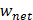# A particle which is experiencing a force, given by=312undergoes a displacement of=4. If the particle had a kinetic energy of 3 J at the beginning of the displacement, what is its kinetic energy at the end of the displacement? (a) 15 J (b) 10 J (c) 12 J (d) 9 J

## Question ID - 50285 :- A particle which is experiencing a force, given by=312undergoes a displacement of=4. If the particle had a kinetic energy of 3 J at the beginning of the displacement, what is its kinetic energy at the end of the displacement? (a) 15 J (b) 10 J (c) 12 J (d) 9 J

3537

Work done ==12J

Work energy theorem=12=3

Kf =15J

Next Question :
 The wheatstone bridge showing in Fig. Here, gets balanced when the carbon resistor used as R1 has the colour code (Orange, Red, Brown). The resistors R 2 and R4 are 80and 40, respectively. Assuming that the colour code for the carbon resistors gives their accurate values, the colour code for the carbon resistor, used as R3 would be:(a) Red, Green, Brown (b) Brown, Blue, Brown (c) Grey, Black, Brown (d) Brown, Blue, Black